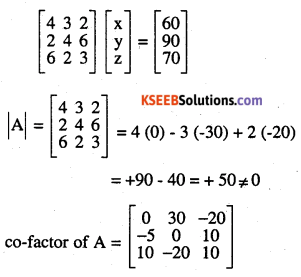# 2nd PUC Maths Question Bank Chapter 4 Determinants Ex 4.6

Students can Download Maths Chapter 4 Determinants Ex 4.6 Questions and Answers, Notes Pdf, 2nd PUC Maths Question Bank with Answers helps you to revise the complete Karnataka State Board Syllabus and score more marks in your examinations.

## Karnataka 2nd PUC Maths Question Bank Chapter 4 Determinants Ex 4.6

### 2nd PUC Maths Determinants NCERT Text Book Questions and Answers Ex 4.6

Examine the consistency of the system of equations.

Question 1.
x + 2y = 2
2x + 3y =2hence consistence and unique solution.

Question 2.
2x – y = 5
x + y = 4hence system is consistent and has unique solution

Question 3.
x + 3y = 5
2x + 6y = 8Question 4.
x + y + z = 1
2x + 3y + 22 = 2
ax + ay + 2az = 4case (i).
If a ≠ 0,
The system is consistent and unique solution
case ii. If a = 0  we can’t say any thing about
consistency
Now we find whether
(Adj A) B is zero or not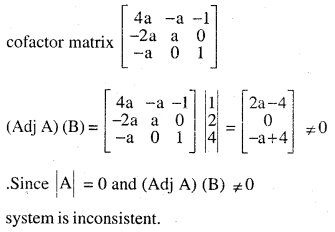Question 5.
3x – y – 2z = 2
2y – z = -1
3x – 5y = 3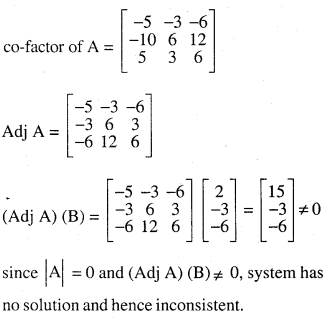Question 6.
5x – y + 4z = 5
2x + 3y + 5z = 2
5x – 2y + 6z = -1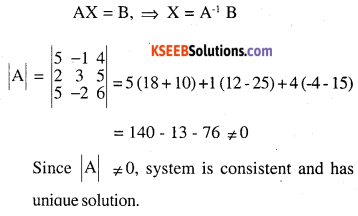Solve the system of linear equations (7 – 14)

Question 7.
5x + 2y = 4
7x + 3y = 5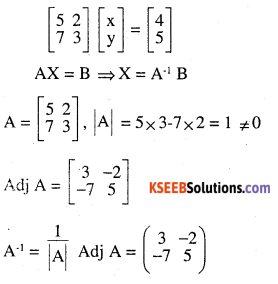Question 8.
2x – y = -2
3x + 4y = 3Question 9.
4x – 3y = 3
3x – 5y = 7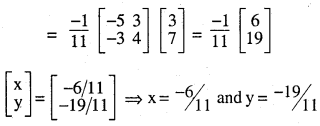Question 10.
5x + 2y = 3
3x + 2y = 5Question 11.
2x + y + z = 1
x – 2y – z = $$\frac{3}{2}$$
3v – 5z =9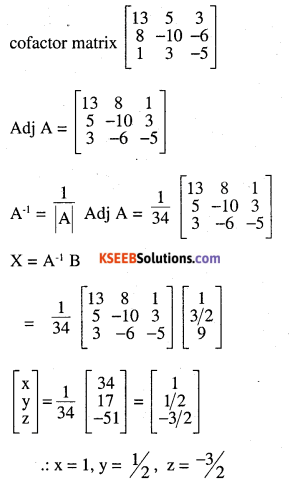Question 12.
x – y + z = 4
2x + y – 3z = 0
x + y + z = 2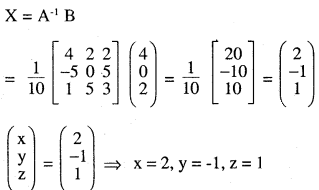Question 13.
2x + 3y + 3z = 5
x – 2y + z = -4
3x – y – 22 = 3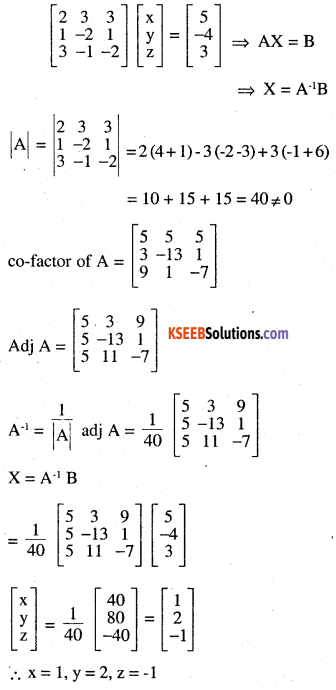Question 14.
x – y + 2z = 7
3x + 4y – 5z = -5
2x – y + 3z = 12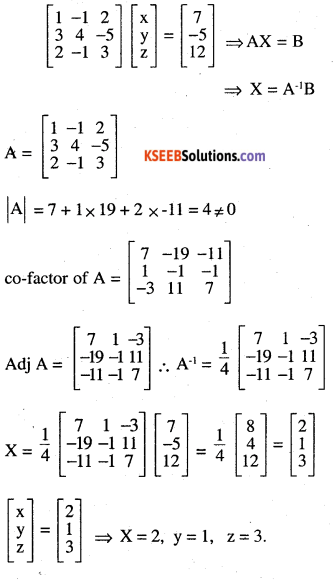Question 15.
$$A=\left[\begin{array}{ccc}{2} & {-3} & {5} \\{3} & {2} & {-4} \\{1} & {1} & {-2}\end{array}\right]$$ find A-1 using A-1 solve
2x – 3y + 5z = 11
3x + 2y – 4z = -5
x + y – 2z = -3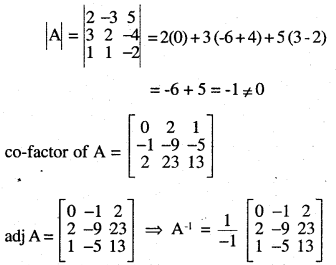Question 16.
The cost of 4 kg onion, 3kg wheat and 2 kg rice is 60/-
The cost of 2 kg onion, 4 kg wheat and 6 kg rice is 90/-­
The cost of 6 kg onion, 2kg wheat and 3kg rice is 70/-
Find the cost of each item per kg by matrix method.